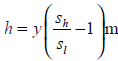# Pressure drop of flowing through a pipe (density 1000 kg/m^3 ) between two points is  measured by using a vertical U-tube manometer. Manometer uses a liquid with density  2000 kg/m^3. The difference in height of manometric liquid in the two limbs of the  manometer is observed to be 10 cm.The pressure drop between the two points.

Question-AnswerCategory: Fluid MechanicsPressure drop of flowing through a pipe (density 1000 kg/m^3 ) between two points is  measured by using a vertical U-tube manometer. Manometer uses a liquid with density  2000 kg/m^3. The difference in height of manometric liquid in the two limbs of the  manometer is observed to be 10 cm.The pressure drop between the two points.

### Pressure drop of flowing through a pipe (density 1000 kg/m^3 ) between two points is  measured by using a vertical U-tube manometer. Manometer uses a liquid with density  2000 kg/m^3. The difference in height of manometric liquid in the two limbs of the  manometer is observed to be 10 cm.The pressure drop between the two points.of light fluid or h = 0.1= 0.1m of light fluid